Evaluate the following limit, L=

# Evaluate the following limit, L=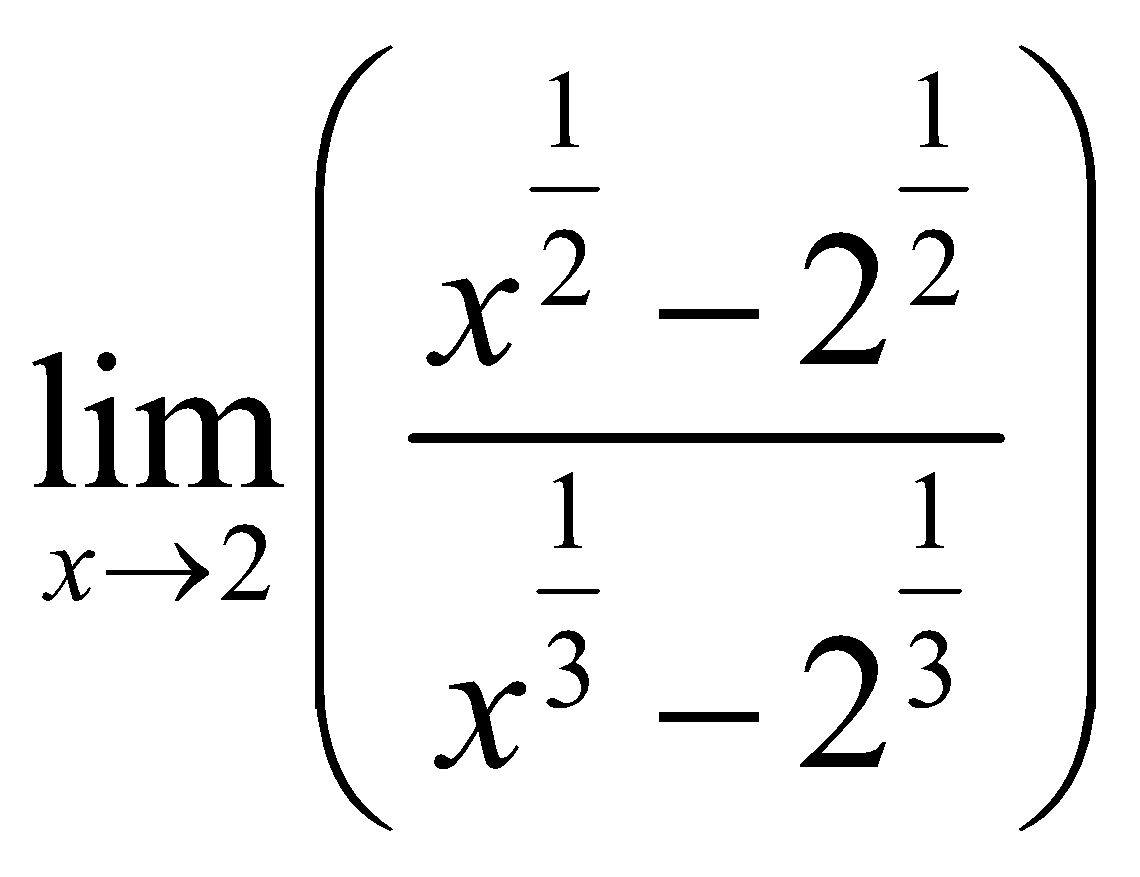1. A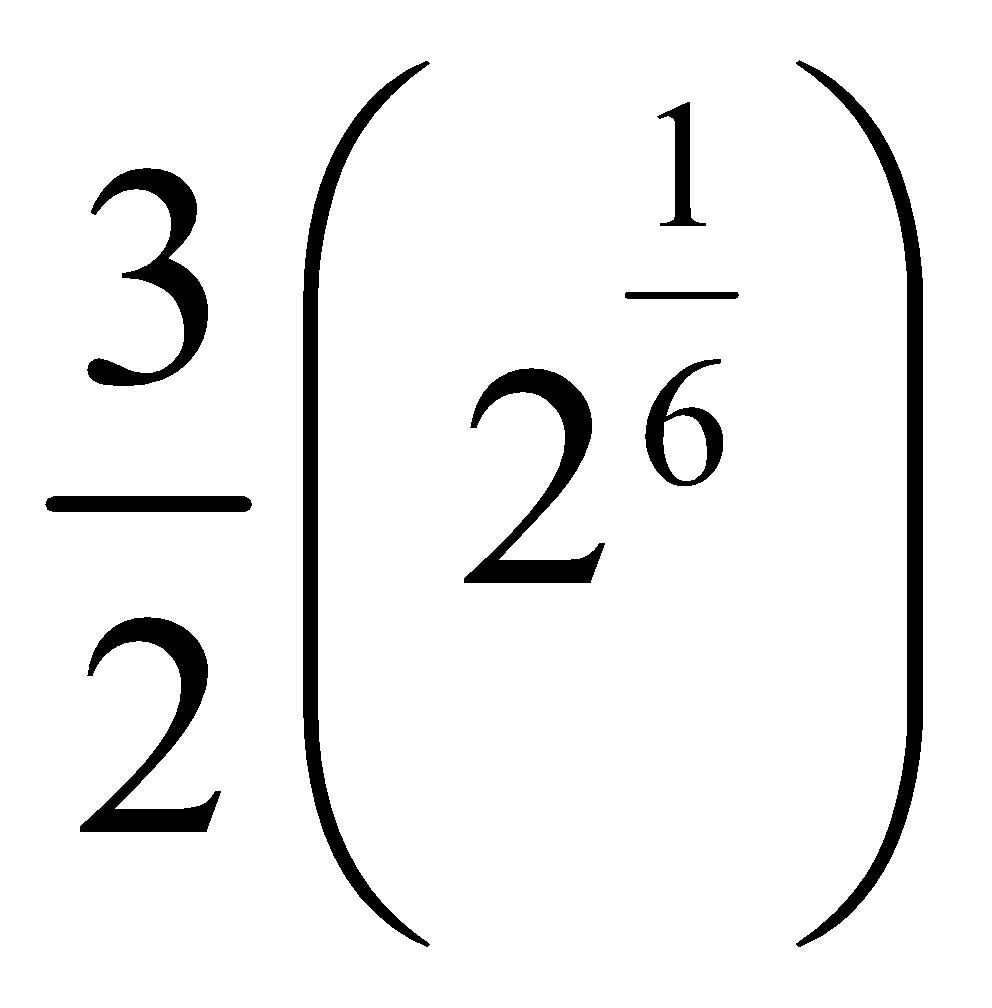2. B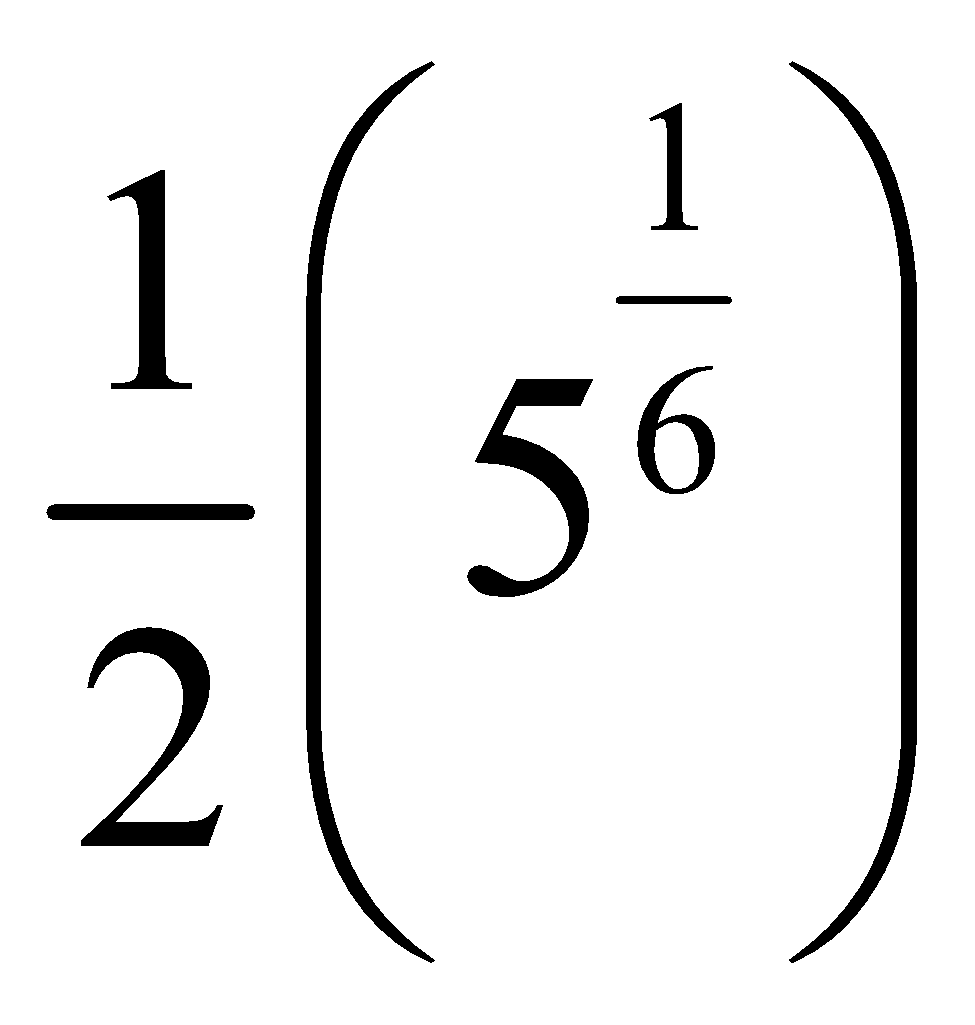3. C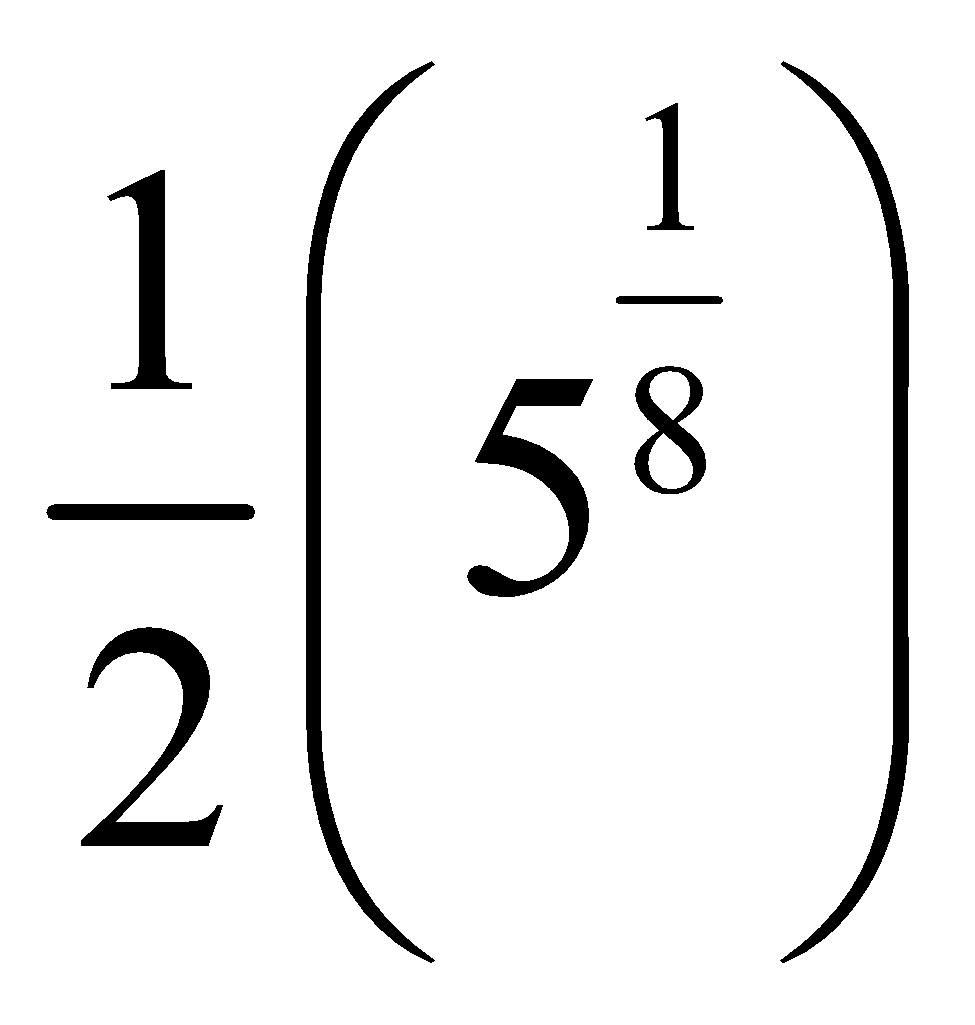4. D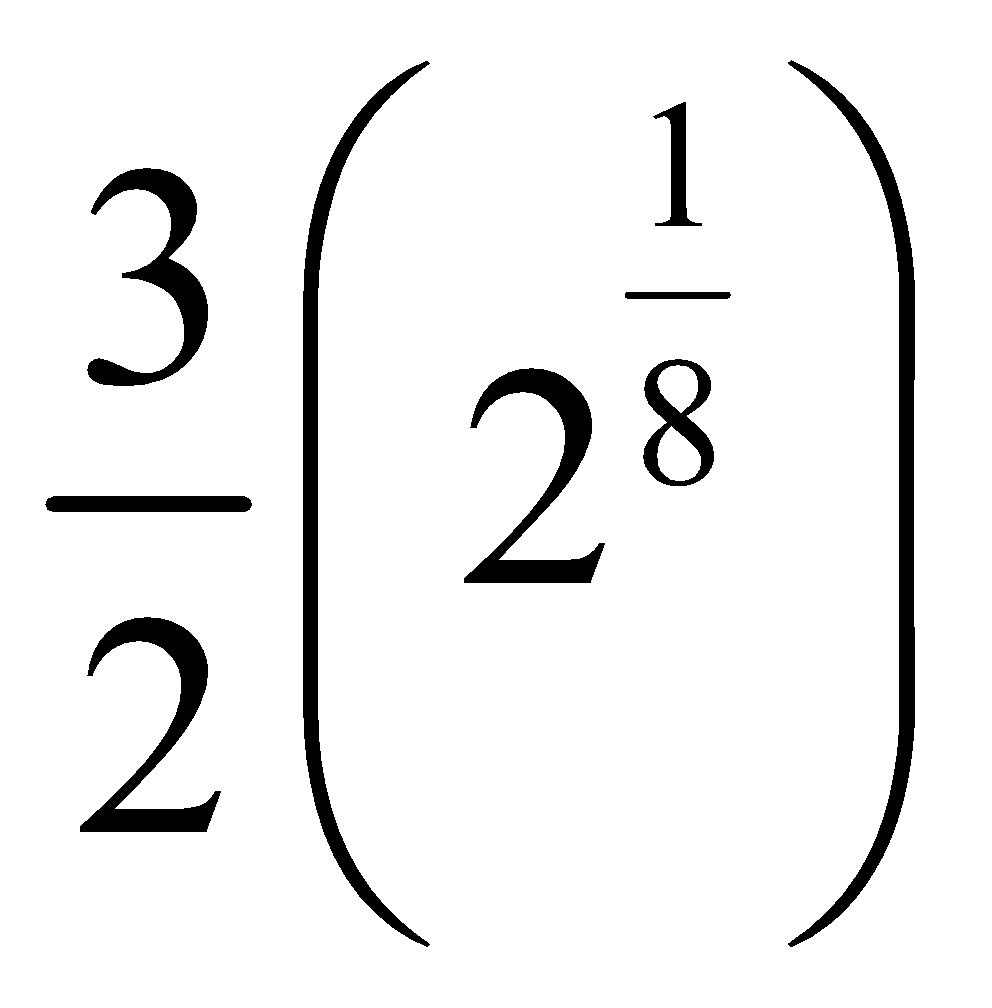Fill Out the Form for Expert Academic Guidance!l

+91

Live ClassesBooksTest SeriesSelf Learning

Verify OTP Code (required)

### Solution:

We have given, L =Now, First multiply and divide the numerator and denominator by, x-2
So we get, L =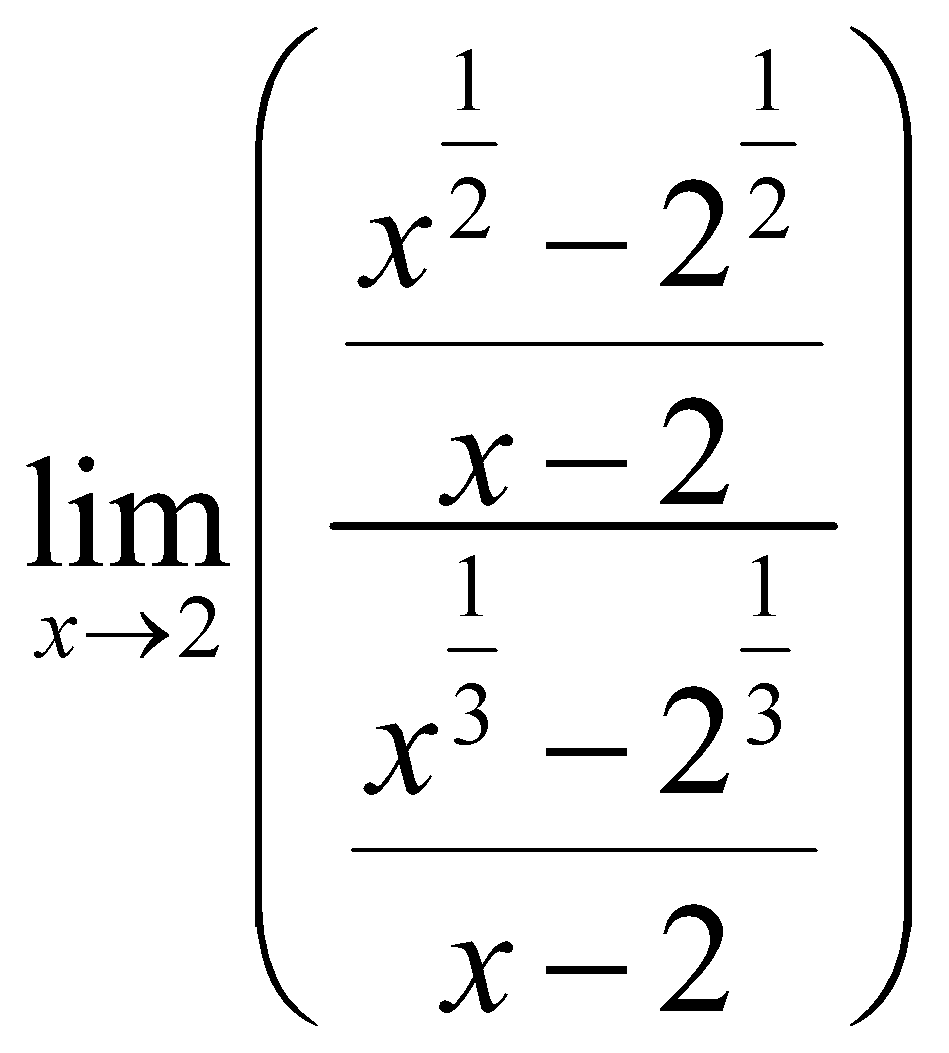Separate the limit to the both numerator and the denominator, we get
L=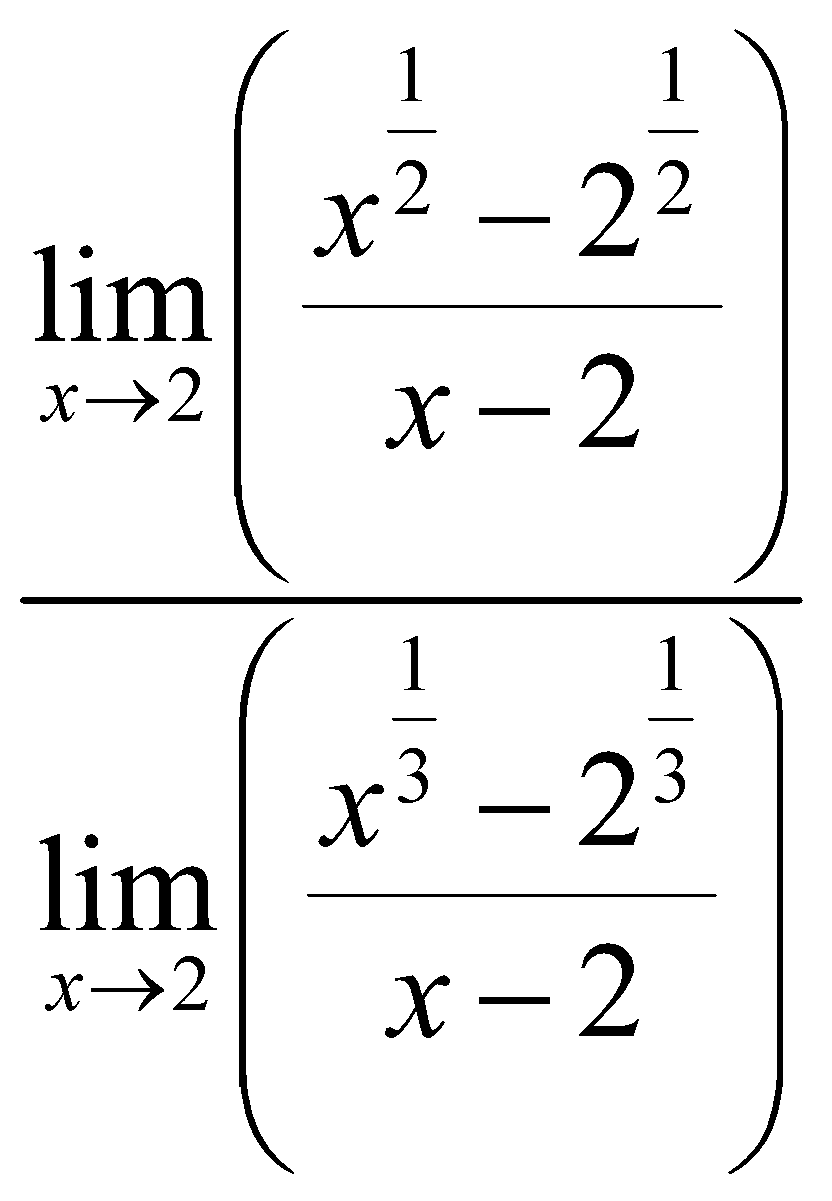Now apply the formula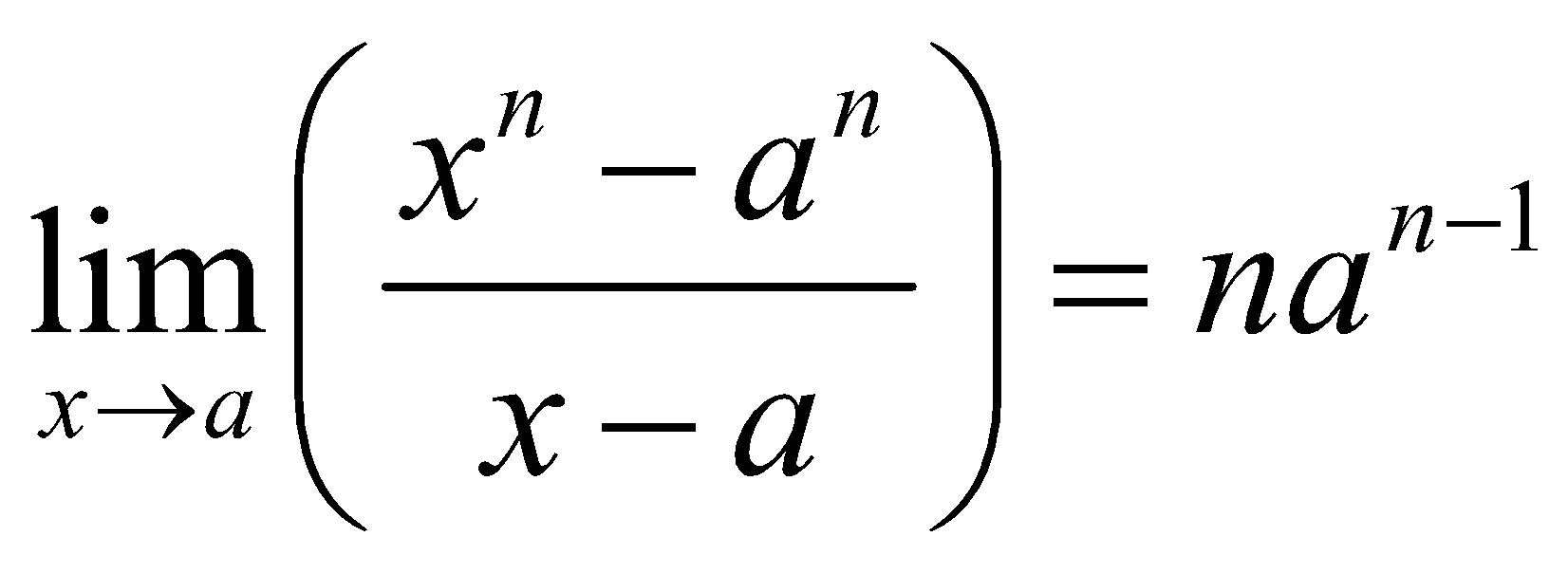to the numerator and denominator both,
So we get, L =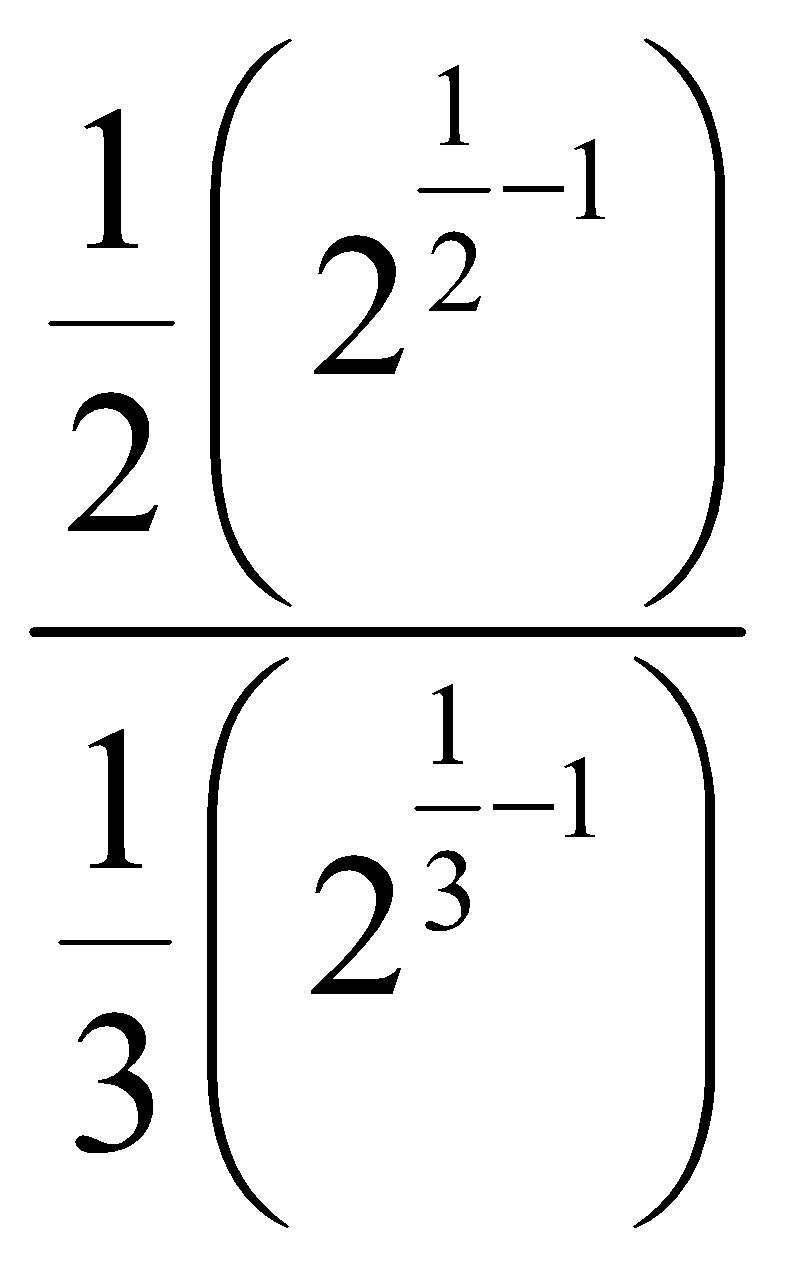Further solving we get,
L =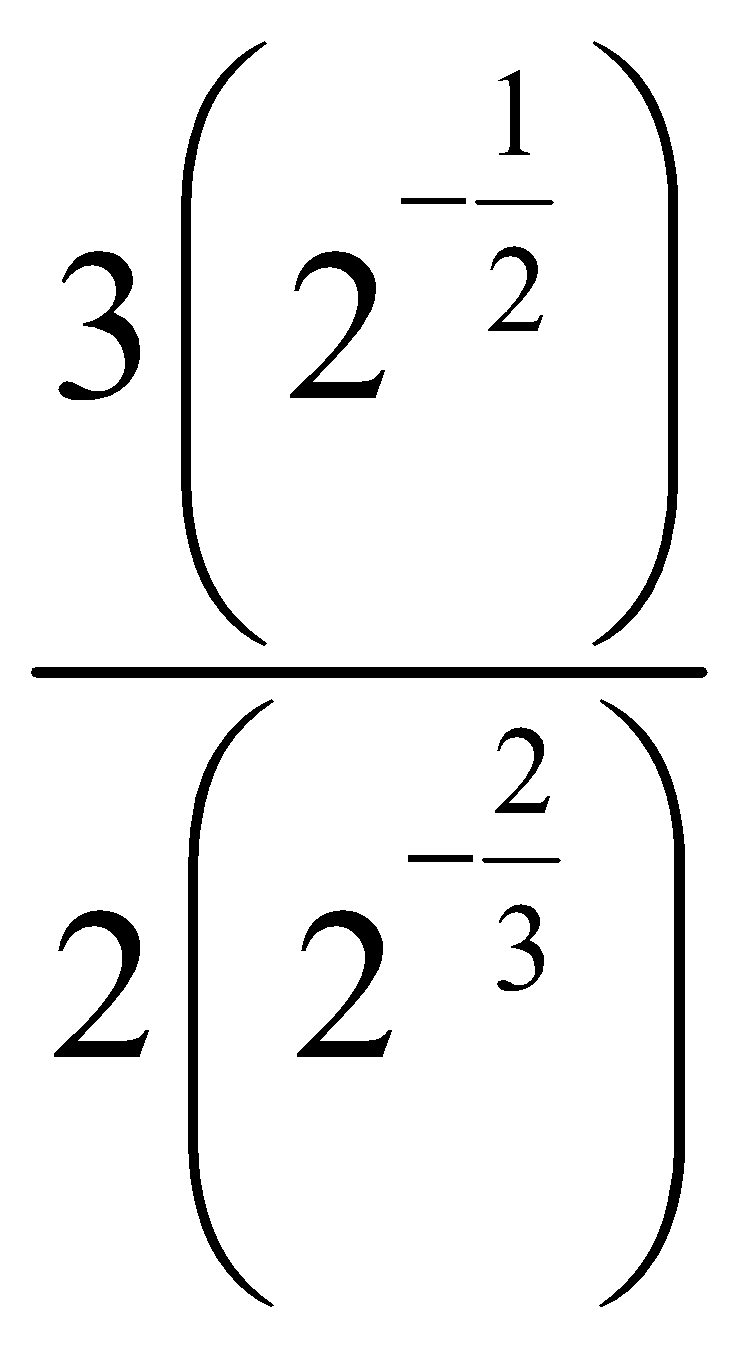(from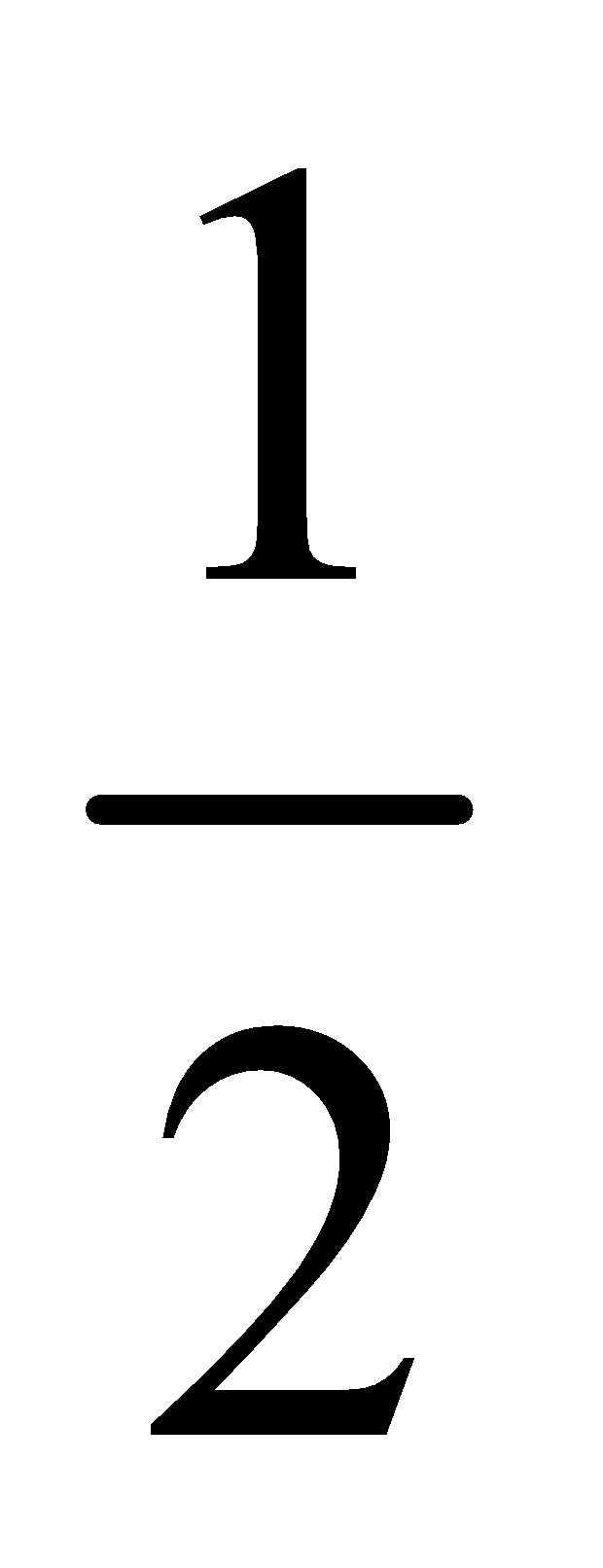the 2 multiplies in to the denominator and in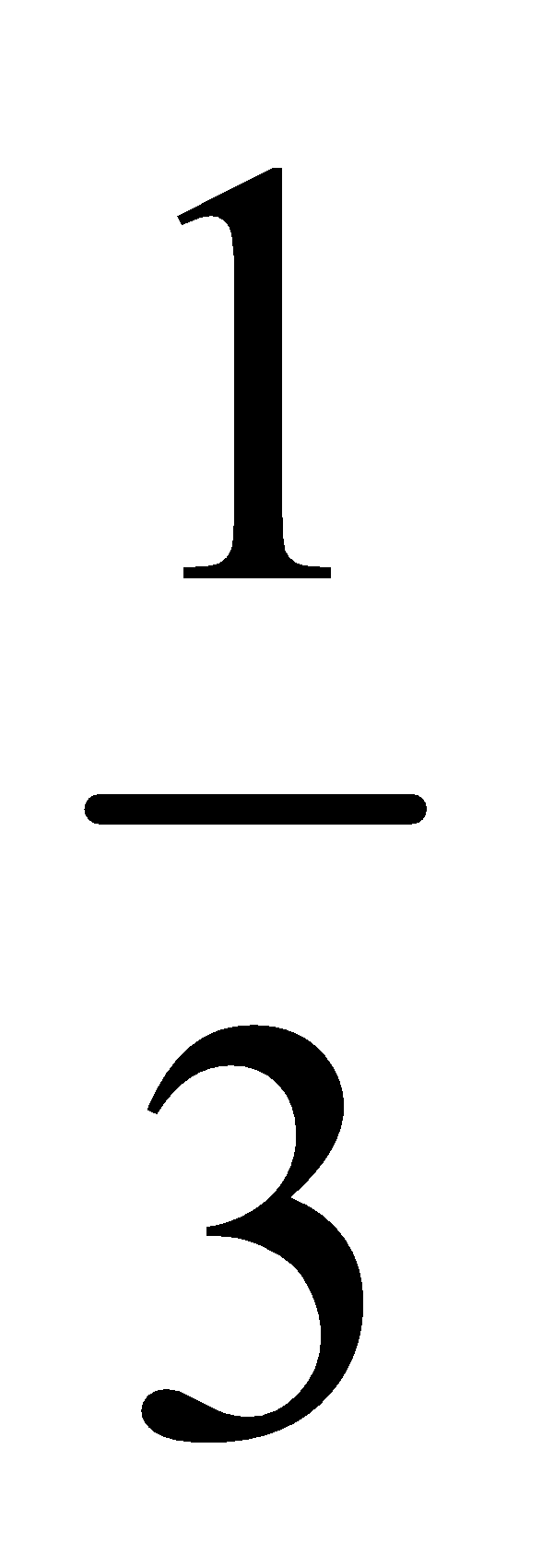the 3 goes into the numerator)
By applying laws of the indices we get,
L=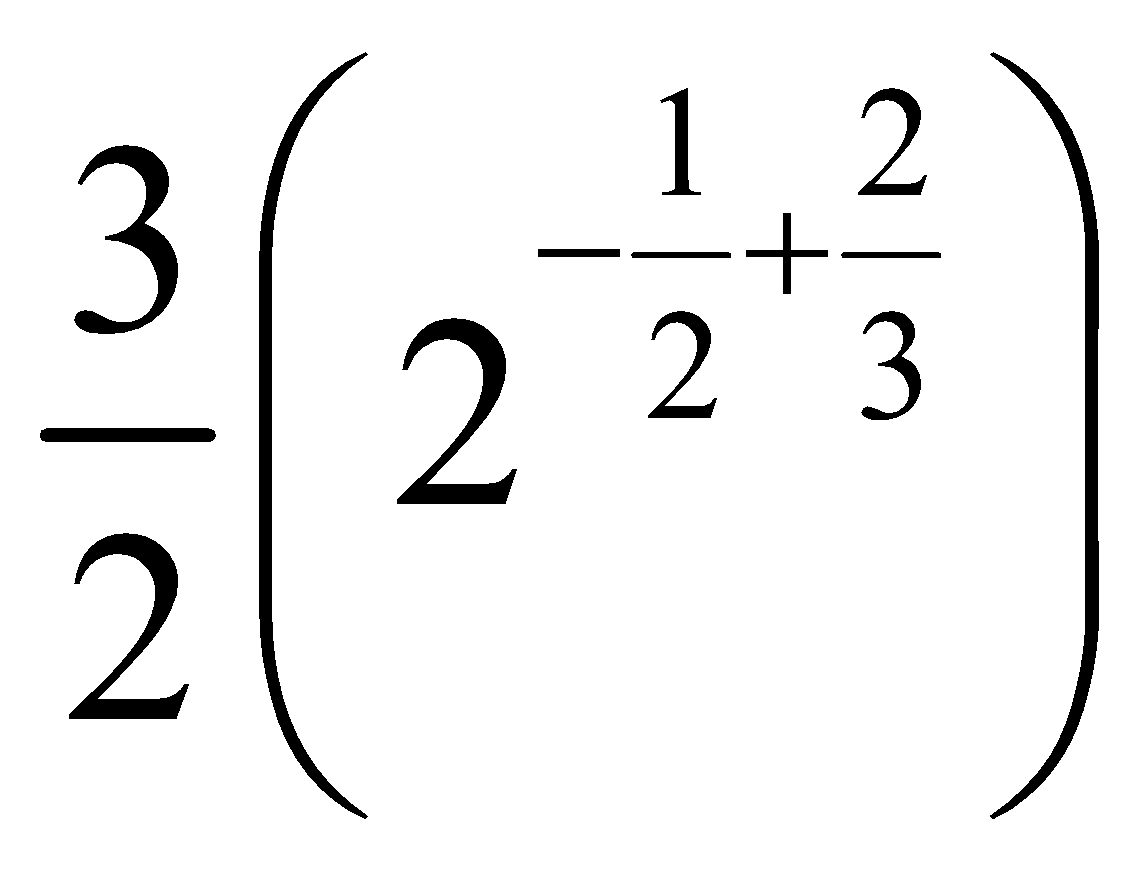L =Therefore our final value of the given limit is L =So, option 1 is correct.

## Related content

 Area of Square Area of Isosceles Triangle Pythagoras Theorem Triangle Formula Perimeter of Triangle Formula Area Formulae Volume of Cone Formula Matrices and Determinants_mathematics Critical Points Solved Examples Type of relations_mathematics+91

Live ClassesBooksTest SeriesSelf Learning

Verify OTP Code (required)